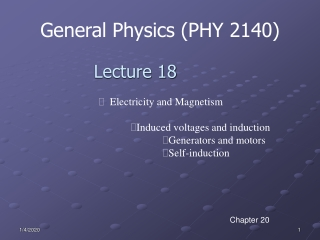DownloadDownload PresentationGeneral Physics (PHY 2140)

# General Physics (PHY 2140)

Télécharger la présentation## General Physics (PHY 2140)

- - - - - - - - - - - - - - - - - - - - - - - - - - - E N D - - - - - - - - - - - - - - - - - - - - - - - - - - -
##### Presentation Transcript

1. General Physics (PHY 2140) Lecture 18 • Electricity and Magnetism • Induced voltages and induction • Generators and motors • Self-induction Chapter 20

2. AC Generators • Basic operation of the generator • As the loop rotates, the magnetic flux through it changes with time • This induces an emf and a current in the external circuit • The ends of the loop are connected to slip rings that rotate with the loop • Connections to the external circuit are made by stationary brushed in contact with the slip rings

3. AC generator (cont) • Generalize the result to N loops where we also noticed that A=la • Note: is reached when wt=90˚ or 270˚ (loop parallel to the magnetic field) EMF generated by the AC generator

4. DC generator • By a clever change to the rings and brushes of the ac generator, we can create a dc generator, that is, a generator where the polarity of the emf is always positive. • The basic idea is to use a single split ring instead of two complete rings. The split ring is arranged so that, just as the emf is about to change sign from positive to negative, the brushes cross the gap, and the polarity of the contacts is switched. • The polarity of the contacts changes in phase with the polarity of the emf -- the two changes essentially cancel each other out, and the emf remains always positive. • The emf still varies sinusoidally during each half cycle, but every half cycle is a positive emf.

5. Motors • A motor is basically a generator running in reverse. A current is passed through the coil, producing a torque and causing the coil to rotate in the magnetic field. Once turning, the coil of the motor generates a back emf, just as does the coil of a generator. The back emf cancels some of the applied emf, and limits the current through the coil.

6. Motors and Back emf • The phrase back emf is used for an emf that tends to reduce the applied current • When a motor is turned on, there is no back emf initially • The current is very large because it is limited only by the resistance of the coil

7. 20.7 Self-inductance • When a current flows through a loop, the magnetic field created by that current has a magnetic flux through the area of the loop. • If the current changes, the magnetic field changes, and so the flux changes giving rise to an induced emf. This phenomenon is called self-induction because it si the loop's own current, and not an external one, that gives rise to the induced emf. • Faraday’s law states

8. The magnetic flux is proportional to the magnetic field, which is proportional to the current in the circuit • Thus, the self-induced EMF must be proportional to the time rate of change of the current where L is called the inductance of the device • Units: SI: henry (H) • If flux is initially zero,

9. Example: solenoid A solenoid of radius 2.5cm has 400 turns and a length of 20 cm. Find (a) its inductance and (b) the rate at which current must change through it to produce an emf of 75mV.

10. 20.9 Energy stored in a magnetic field • The battery in any circuit that contains a coil has to do work to produce a current • Similar to the capacitor, any coil (or inductor) would store potential energy